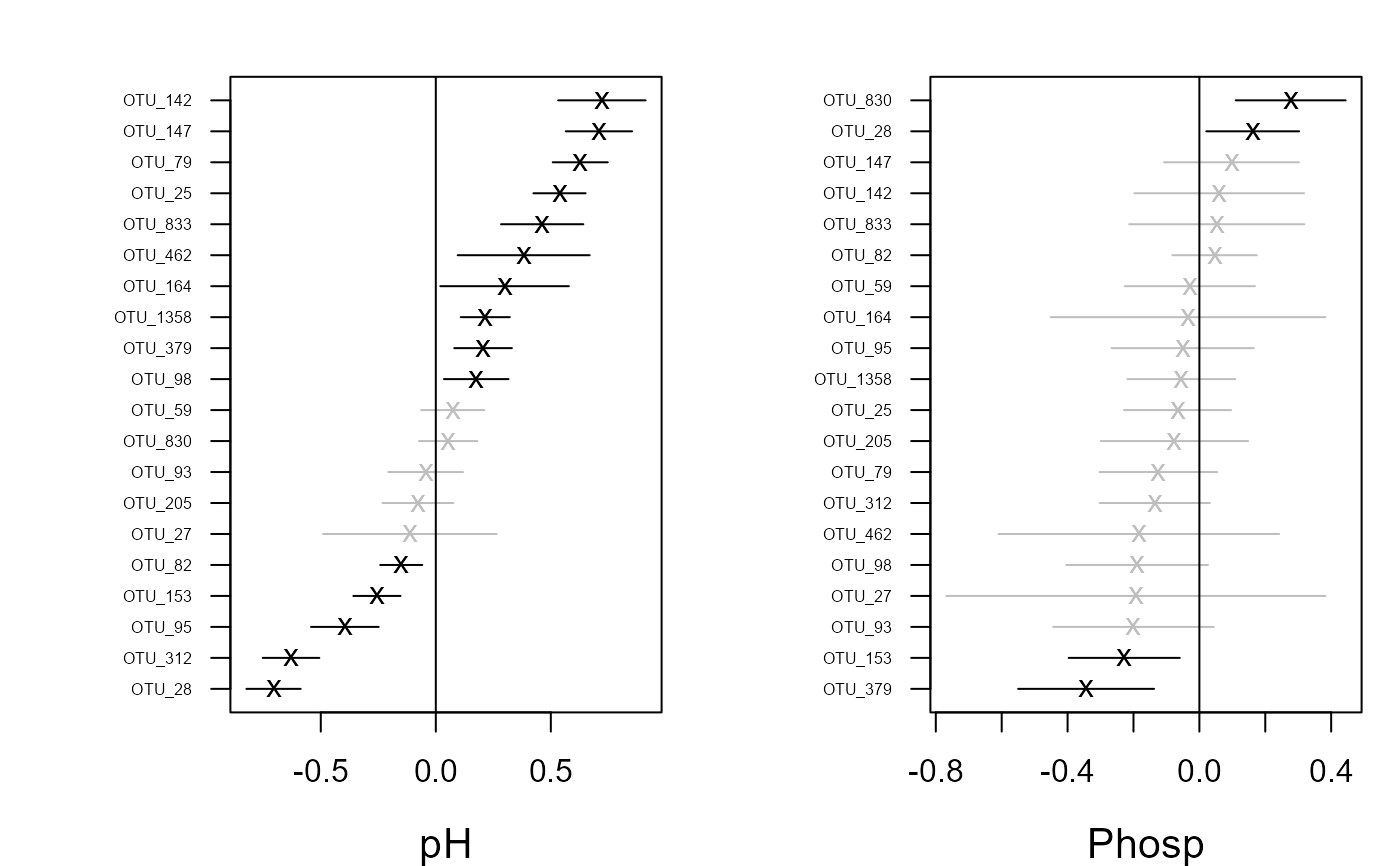Plots covariate coefficients and their confidence intervals.

# S3 method for gllvm
coefplot(
object,
y.label = TRUE,
which.Xcoef = NULL,
order = TRUE,
cex.ylab = 0.5,
mfrow = NULL,
mar = c(4, 6, 2, 1),
xlim.list = NULL,
...
)

## Arguments

object an object of class 'gllvm'. logical, if TRUE (default) colnames of y with respect to coefficients are added to plot. vector indicating which covariate coefficients will be plotted. Can be vector of covariate names or numbers. Default is NULL when all covariate coefficients are plotted. logical, whether or not coefficients are ordered, defaults to TRUE. the magnification to be used for axis annotation relative to the current setting of cex. same as mfrow in par. If NULL (default) it is determined automatically. vector of length 4, which defines the margin sizes: c(bottom, left, top, right). Defaults to c(4,5,2,1). list of vectors with length of two to define the intervals for an x axis in each covariate plot. Defaults to NULL when the interval is defined by the range of point estimates and confidence intervals additional graphical arguments.

## Author

Jenni Niku <jenni.m.e.niku@jyu.fi>, Francis K.C. Hui, Sara Taskinen

## Examples

# Extract subset of the microbial data to be used as an example
data(microbialdata)
X <- microbialdata$Xenv y <- microbialdata$Y[, order(colMeans(microbialdata$Y > 0), decreasing = TRUE)[21:40]] fit <- gllvm(y, X, formula = ~ pH + Phosp, family = poisson()) coefplot(fit)if (FALSE) { ## Load a dataset from the mvabund package data(antTraits) y <- as.matrix(antTraits$abund)
X <- as.matrix(antTraits$env) # Fit model with environmental covariates fit <- gllvm(y, X, formula = ~ Bare.ground + Shrub.cover, family = poisson()) coefplot.gllvm(fit) # Fit model with all environmental covariates fitx <- gllvm(y, X, family = "negative.binomial") coefplot(fitx, mfrow = c(3,2)) coefplot(fitx, which.Xcoef = 1:2) # Fit gllvm model with environmental and trait covariates TR <- antTraits$traits
fitT <- gllvm(y = y, X = X, TR = TR, family = "negative.binomial")
coefplot(fitT)
}